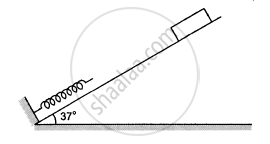Advertisement Remove all ads

# Figure Following Shows a Spring Fixed at the Bottom End of an Incline of Inclination 37°. a Small Block of Mass 2 Kg Starts Slipping Down the Incline from a Point 4⋅8 M Away from the Spring. - Physics

Numerical

Following figure shows a spring fixed at the bottom end of an incline of inclination 37°. A small block  of mass 2 kg starts slipping down the incline from a point 4⋅8 m away from the spring. The block compresses the spring by 20 cm, stops momentarily and then rebounds through a distance of 1 m up the incline. Find (a) the friction coefficient between the plane and the block and (b) the spring constant of the spring. Take g = 10 m/s2.Advertisement Remove all ads

#### Solution

$\text{ Given: }$
$\text{ Mass of the block, m = 2 kg }$
$\text{ Initial distance of the block from the spring , S}_1 = 4 . 8 \text{m},$
$\text{ Comression in the spring, x = 20 cm = 0 . 2 m }$
$\text{ Final distance of the block from the spring, S} _2 = 1 \text{ m }$
$\text{ As }\theta = 37^\circ,$
$\sin 37^\circ= 0 . 60 = \frac{3}{5}$
$\cos 37^\circ= 0 . 80 = \frac{4}{5}$

Applying the work-energy principle for downward motion of the block,

$0 - 0 = \text{mg } \sin 37^\circ\left( \text{ x} + 4 . 8 \right) - \mu \text{ R } \times 5 - \frac{1}{2}\text{ kx}^2$
$\Rightarrow 20 \times \left( 0 . 06 \right) \times 5 - \mu \times 20 \times \left( 0 . 80 \right) \times 5 - \frac{1}{2}\text{k} \left( 0 . 2 \right)^2 = 0$
$\Rightarrow 60 - 80 \mu - 0 . 02 \text{ k } = 0$
$\Rightarrow 80 \mu + 0 . 02 \text{k = 60 . . . (i)}$

Similarly for the upward motion of the body the equation is

$0 - 0 = \left( - \text{ mg } \sin 37^\circ \right) - \mu \text{ R } \times 1 + \frac{1}{2}\text{ k } \left( - 2 \right)^2$

$\Rightarrow 20 \times \left( 0 . 06 \right) \times 1 - \mu \times 20 \times \left( 0 . 80 \right) \times 1 - \frac{1}{2}\text{ k } \left( 0 . 2 \right)^2$

$\Rightarrow 12 - 16 \mu + 0 . 02 \text{ k } = 0$

Adding equations (i) and (ii), we get:

$96 \mu = 48$
$\Rightarrow \mu = 0 . 5$

Now putting the value of μ  in equation (i), we get:
k = 1000 N/m

Is there an error in this question or solution?
Advertisement Remove all ads

#### APPEARS IN

HC Verma Class 11, 12 Concepts of Physics 1
Chapter 8 Work and Energy
Q 43 | Page 135
Advertisement Remove all ads
Advertisement Remove all ads
Share
Notifications

View all notifications

Forgot password?